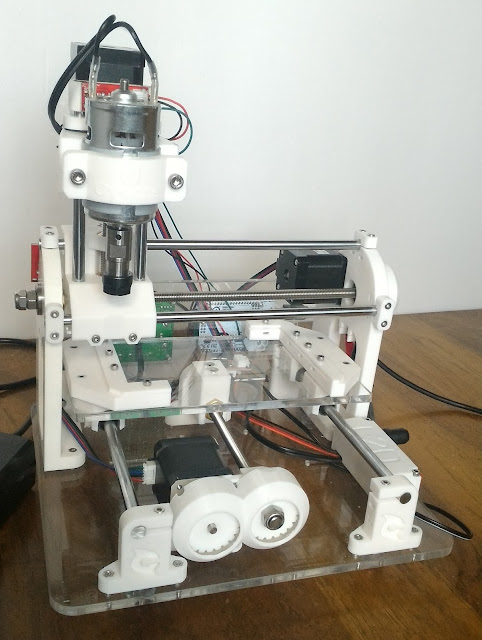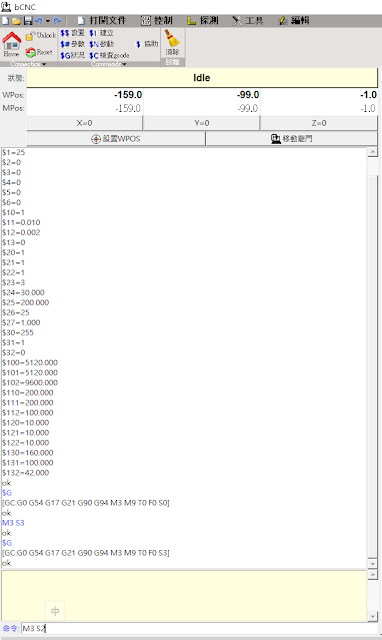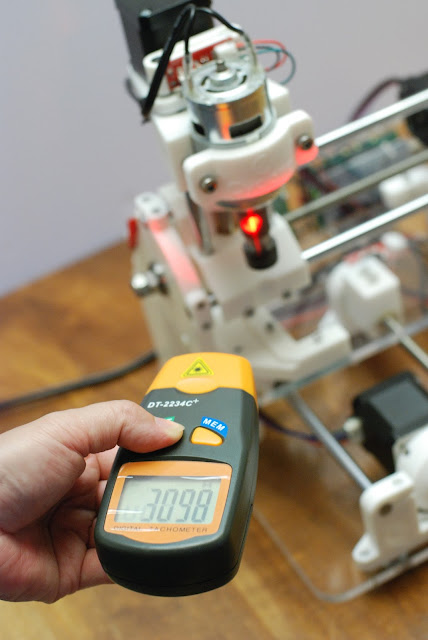## 2017年8月4日 星期五

### GRBL 非線性主軸速度PWM輸出解決方案

最近重組了一台白色的cyclone PCB，才發現之前所撰寫的那一篇文章很多資料都不是很清楚，所以同好遇許多問題，所以如果有問題就提出來。因為我一開始也不是很清楚，只是透過網路參考之組裝過的同好所撰寫的文章，組裝過程不懂的地方也一直發問。重組了一台白色的cyclone PCB

所以新的技術就是要發表新的文章，這一篇主要討論的事　＂GRBL 非線性主軸速度PWM輸出解決方案＂ Open Source的最大好處就是擁有全世界的工程師協助解決問題並提出創新的技術．那真的是件很棒的事情。

什麼是＂非線性主軸速度PWM輸出解決方案＂因為我們的主軸控制使用PWM輸出，所以輸出曲線是一個線性曲線（０％～１００％再依我們主軸最大轉速去換算），我們的主軸並不是這麼的線性，簡單的說我們的主軸馬達轉速有10000轉，而PWM設定50%輸出，照道理講主軸馬達轉速應該輸5000轉，但你實際量測馬達轉速只達到3000，所以為了避免這個問題發生，所以就有人提出了非線性主軸函式。

這非線性主軸函式是　麻省理工學院許可證（MIT）Copyright (c) 2017 Sungeun K. Jeon for 怎麼做呢？　我們需要一個硬體設備。就是轉速計，不管是接觸式和非接觸式的只要能測量出你的主軸真實轉速，就可以做出非線性主軸轉速控制。

而且在這個＂非線性主軸速度PWM輸出解決方案＂是GRBL v1.1f.20170717 才有的功能所以你不要下載太舊的版本。文件中含有使用說明

 +USAGE: + - First, make sure you are using the stock build of Grbl for the 328p processor. Most + importantly, the SPINDLE_PWM_MAX_VALUE and SPINDLE_PWM_MIN_VALUE should be unaltered + from defaults, otherwise change them back to 255.0 and 1.0 respectively for this test. + + - Next, program the max and min rpm Grbl settings to '\$30=255' and '\$31=1'. This sets + the internal PWM values equal to 'S' spindle speed for the standard Grbl build. + + - Check if your spindle does not turn on at very low voltages by setting 'S' spindle + speed to 'S1'. If it does not turn on or turns at a non-useful rpm, increase 'S' by + one until it does. Write down this 'S' value for later. You'll start the rpm data + collection from this point onward and will need to update the SPINDLE_PWM_MIN_VALUE + in cpu_map.h afterwards. + + - Collect actual spindle speed with a tachometer or similar means over a range of 'S' + and PWM values. Start by setting the spindle 'S' speed to the minimum useful 'S' from + the last step and measure and record actual spindle rpm. Next, increase 'S' spindle + speed over equally sized intervals and repeat the measurement. Increments of 20 rpm + should be more than enough, but decrease increment size, if highly nonlinear. Complete + the data collection the 'S' spindle speed equal to '\$30' max rpm, or at the max useful + rpm, and record the actual rpm output. Make sure to collect rpm data all the way + throughout useful output rpm. The actual operating range within this model may be set + later within Grbl with the '\$30' and '\$31' settings. + + - In some cases, spindle PWM output can have discontinuities or not have a useful rpm + in certain ranges. For example, a known controller board has the spindle rpm drop + completely at voltages above ~4.5V. If you have discontinuities like this at the low + or high range of rpm, simply trim them from the data set. Don't include them. For + Grbl to compensate, you'll need to alter the SPINDLE_PWM_MIN_VALUE and/or + SPINDLE_PWM_MAX_VALUE in cpu_map.h to where your data set ends. This script will + indicate if you need to do that in the solution output. + + - Keep in mind that spindles without control electronics can slow down drastically when + cutting and under load. How much it slows down is dependent on a lot of factors, such + as feed rate, chip load, cutter diameter, flutes, cutter type, lubricant/coolant, + material being cut, etc. Even spindles with controllers can still slow down if the + load is higher than the max current the controller can provide. It's recommended to + frequently re-check and measure actual spindle speed during a job. You can always use + spindle speed overrides to tweak it temporarily to the desired speed. + + - Edit this script and enter the measured rpm values and their corresponding 'S' spindle + speed values in the data arrays below. Set the number of piecewise lines you would + like to use, from one to four lines. For most cases, four lines is perfectly fine. + In certain scenarios (laser engraving), this may significantly degrade performance and + should be reduced if possible. + + - Run the Python script. Visually assess the line fit from the plot. It will not likely + to what you want on the first go. Dial things in by altering the line fit junction + points 'PWM_pointX' in this script to move where the piecewise line junctions are + located along the plot x-axis. It may be desired to tweak the junction points so the + model solution is more accurate in the region that the spindle typically running. + Re-run the script and tweak the junction points until you are satified with the model. + + - Record the solution and enter the RPM_POINT and RPM_LINE values into config.h. Set the + number of piecewise lines used in this model in config.h. Also set the '\$30' and '\$31' + max and min rpm values to the solution values or in a range between them in Grbl '\$' + settings. And finally, alter the SPINDLE_PWM_MIN_VALUE in cpu_map.h, if your spindle + needs to be above a certain voltage to produce a useful low rpm. + + - Once the solution is entered. Recompile and flash Grbl. This solution model is only + valid for this particular set of data. If the machine is altered, you will need to + perform this experiment again and regenerate a new model here. + +OUTPUT: + The solver produces a set of values that define the piecewise fit and can be used by + Grbl to quickly and efficiently compute spindle PWM output voltage for a desired RPM. + + The first two are the RPM_MAX (\$30) and RPM_MIN (\$31) Grbl settings. These must be + programmed into Grbl manually or setup in defaults.h for new systems. Altering these + values within Grbl after a piece-wise linear model is installed will not change alter + model. It will only alter the range of spindle speed rpm values Grbl output. + + For example, if the solver produces an RPM_MAX of 9000 and Grbl is programmed with + \$30=8000, S9000 may be programmed, but Grbl will only produce the output voltage to run + at 8000 rpm. In other words, Grbl will only output voltages the range between + max(RPM_MIN,\$31) and min(RPM_MAX,\$30). + + The remaining values define the slopes and offsets of the line segments and the junction + points between line segments, like so for n_pieces=3: + + PWM_output = RPM_LINE_A1 * rpm - RPM_LINE_B1 [ RPM_MIN < rpm < RPM_POINT12 ] + PWM_output = RPM_LINE_A2 * rpm - RPM_LINE_B2 [ RPM_POINT12 < rpm < RPM_POINT23 ] + PWM_output = RPM_LINE_A3 * rpm - RPM_LINE_B3 [ RPM_POINT23 < rpm < RPM_MAX ] + + NOTE: The script solves in terms of PWM but the final equations and values are expressed + in terms of rpm in the form 'PWM = a*rpm - b'. + +""" + +from scipy import optimize +import numpy as np + +# ---------------------------------------------------------------------------------------- +# Configure spindle PWM line fit solver + +n_pieces = 4 # Number of line segments used for data fit. Only 1 to 4 line segments supported. + +# Programmed 'S' spindle speed values. Must start with minimum useful PWM or 'S' programmed +# value and end with the maximum useful PWM or 'S' programmed value. Order of the array must +# be synced with the RPM_measured array below. +# NOTE: ** DO NOT USE DATA FROM AN EXISTING PIECEWISE LINE FIT. USE DEFAULT GRBL MODEL ONLY. ** +PWM_set = np.array([2,18,36,55,73,91,109,127,146,164,182,200,218,237,254], dtype=float) + +# Actual RPM measured at the spindle. Must be in the ascending value and equal in length +# as the PWM_set array. Must include the min and max measured rpm output in the first and +# last array entries, respectively. +RPM_measured = np.array([213.,5420,7145,8282,9165,9765,10100,10500,10700,10900,11100,11250,11400,11550,11650], dtype=float) + +# Configure line fit points by 'S' programmed rpm or PWM value. Values must be between +# PWM_max and PWM_min. Typically, alter these values to space the points evenly between +# max and min PWM range. However, they may be tweaked to maximize accuracy in the places +# you normally operate for highly nonlinear curves. Plot to visually assess how well the +# solution fits the data. +PWM_point1 = 20.0 # (S) Point between segments 0 and 1. Used when n_pieces >= 2. +PWM_point2 = 80.0 # (S) Point between segments 1 and 2. Used when n_pieces >= 3. +PWM_point3 = 150.0 # (S) Point between segments 2 and 3. Used when n_pieces = 4. + +# ---------------------------------------------------------------------------------------- + +# Advanced settings + +# The optimizer requires an initial guess of the solution. Change value if solution fails. +slope_i = 100.0; # > 0.0 + +PWM_max = max(PWM_set) # Maximum PWM set in measured range +PWM_min = min(PWM_set) # Minimum PWM set in measured range +plot_figure = True # Set to False, if matplotlib is not available. +

PWM_set = np.array([2,18,36,55,73,91,109,127,146,164,182,200,218,237,254], dtype=float)

RPM_measured =np.array([213.,5420,7145,8282,9165,9765,10100,10500,10700,10900,11100,11250,11400,11550,11650],dtype=float)bCNC 命令列上數入 M3 S2測量主軸轉速

PWM_set = np.array([10,18,36,55,73,91,109,127,146,164,182,200,218,237,254], dtype=float)

RPM_measured = np.array([1622,3098,6696,9570,10782,11730,12303,12833,13199,13391,13630,13844,14054,14210,14500], dtype=float)